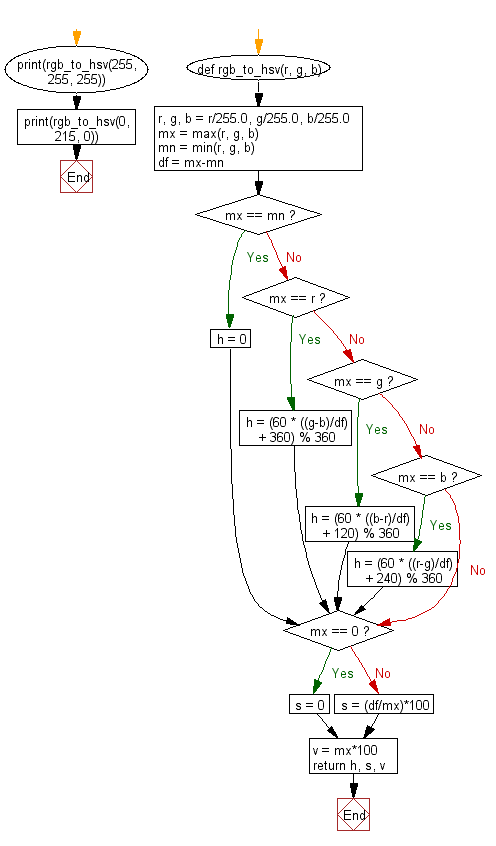﻿ Python Math: Convert RGB color to HSV color - w3resource# Python Math: Convert RGB color to HSV color

## Python Math: Exercise-77 with Solution

Write a Python program to convert RGB color to HSV color.

Note: RGB - RGB (Red, Green, Blue) describes what kind of light needs to be emitted to produce a given color. Light is added together to create form from darkness. RGB stores individual values for red, green and blue. RGB is not a color space, it is a color model. There are many different RGB color spaces derived from this color model, some of which appear below.

HSV - (hue, saturation, value), also known as HSB (hue, saturation, brightness), is often used by artists because it is often more natural to think about a color in terms of hue and saturation than in terms of additive or subtractive color components. HSV is a transformation of an RGB colorspace, and its components and colorimetry are relative to the RGB colorspace from which it was derived.

Sample Solution:-

Python Code:

``````def rgb_to_hsv(r, g, b):
r, g, b = r/255.0, g/255.0, b/255.0
mx = max(r, g, b)
mn = min(r, g, b)
df = mx-mn
if mx == mn:
h = 0
elif mx == r:
h = (60 * ((g-b)/df) + 360) % 360
elif mx == g:
h = (60 * ((b-r)/df) + 120) % 360
elif mx == b:
h = (60 * ((r-g)/df) + 240) % 360
if mx == 0:
s = 0
else:
s = (df/mx)*100
v = mx*100
return h, s, v

print(rgb_to_hsv(255, 255, 255))
print(rgb_to_hsv(0, 215, 0))
```
```

Sample Output:

```(0, 0.0, 100.0)
(120.0, 100.0, 84.31372549019608)
```

Flowchart:## Visualize Python code execution:

The following tool visualize what the computer is doing step-by-step as it executes the said program:

Python Code Editor:

Have another way to solve this solution? Contribute your code (and comments) through Disqus.

What is the difficulty level of this exercise?

Test your Programming skills with w3resource's quiz.

﻿

```>>> students = [{'name': 'John', 'score': 98}, {'name': 'Mike', 'score': 94}, {'name': 'Jennifer', 'score': 99}]